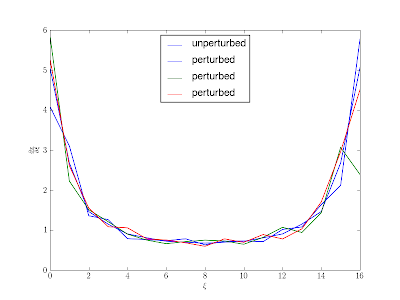## Monday, July 13, 2009

### Derivative of Unequally Spaced Points

I posted a while back on solving Burgers' equation on a moving grid. The same coordinate transformation techniques applied there can be used to calculate the derivative of unequally spaced data points. Just as in the moving grid application, the grid transformation takes the points in the physical space to the computational space. Once in computational space, the sky's the limit!

One way to do this would be just a simple, low-order finite difference for the first derivative, maybe using an average of two one-sided differences (that would reduce to a central difference if the points were equally spaced). We can easily do better than that though since there are so many great, optimized transforms available in the FFTW library. Spectral methods give results that are accurate down to the precision of the machine for more than about 20 points (for functions without discontinuities).

The cost of using a grid-transformation is that we have to calculate two derivatives and then multiply them together, and the cost of a spectral method is O(n*log(n)) instead of O(n) as in a finite difference approximation. In one-dimension things are pretty easy, the chain rule shows us the two derivatives we need to calculate:It is not straight-forward to directly calculate the additional derivative, so we calculate it by inverting the derivative of the physical coordinate with respect to the computational one:Using the discrete cosine transform from FFTW (Chebyshev psuedospectral method) is straightforward to do by using F2Py and Python:

n = 17 # number of data points
a = 0.0 # left side of the interval
b = 3 * np.pi # right side of the interval
alpha = 0.25 # parameter to scale the perturbation
dx = (b - a) / float(n) # this is the equally spaced delta x
half_interval = (b - a) / 2.0 # so the dct diff is properly normalized
n_perturb = 3 # number of perturbed sets to try

dx_perturb = alpha * dx * (sp.random.random_sample((n,n_perturb)) - 0.5)

x = np.linspace(a, b, n)
dxdxi_perturb = np.zeros((n,n_perturb),dtype=float)
dydxi_perturb = np.zeros((n,n_perturb),dtype=float)
y_perturb = np.zeros((n,n_perturb),dtype=float)
x_perturb = np.zeros((n,n_perturb),dtype=float)

for i in xrange(n_perturb):
x_perturb[:,i] = x + dx_perturb[:,i] # add in the random fluctuations

y = np.cos(x)
y_perturb = np.cos(x_perturb)
dxdxi = cs.dct_diff(x, half_interval)
dy_analytical = - np.sin(x)
dydxi = cs.dct_diff(y, half_interval)
# derivatives with respect to the computational coordinate:
for i in xrange(n_perturb):
dxdxi_perturb[:,i] = cs.dct_diff(x_perturb[:,i], half_interval)
dydxi_perturb[:,i] = cs.dct_diff(y_perturb[:,i], half_interval)

# now actually calculate the derivatives we're after:
dydx = dydxi / dxdxi
dydx_perturb = dydxi_perturb / dxdxi_perturb

The derivative of the physical coordinate with respect to the computational coordinate for the equally spaced case and a couple of cases with some random perturbations added:The actual derivative that we're after:This example shows the flexibility of the approach, but it also indicates the importance of smooth changes in grid spacing for accurate derivative approximations. Abrupt changes in grid spacing translates into noise in the derivative.